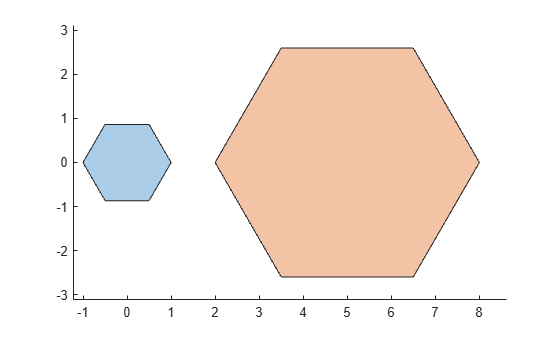# nsidedpoly

Regular polygon

## Syntax

``pgon = nsidedpoly(n)``
``pgon = nsidedpoly(n,Name,Value)``

## Description

example

````pgon = nsidedpoly(n)` returns a regular polygon with `n` equal-length sides. The center of `pgon` is at the point (0,0), and the circumscribed circle of the polygon has radius 1.```

example

````pgon = nsidedpoly(n,Name,Value)` specifies additional properties of the polygon using one or more name-value pair arguments. For example, `pgon = nsidedpoly(4,'SideLength',5)` creates a square centered at (0,0) with sides of length 5.```

## Examples

collapse all

Create a hexagon with sides of length 1 centered at the point (0,0). Then, create a hexagon with sides of length 3 centered at (5,0).

```pgon1 = nsidedpoly(6); pgon2 = nsidedpoly(6,'Center',[5 0],'SideLength',3); plot([pgon1 pgon2]) axis equal```## Input Arguments

collapse all

Number of sides of the polygon, specified as a positive scalar integer greater than 2.

Data Types: `double` | `single` | `int8` | `int16` | `int32` | `int64` | `uint8` | `uint16` | `uint32` | `uint64`

### Name-Value Pair Arguments

Specify optional comma-separated pairs of `Name,Value` arguments. `Name` is the argument name and `Value` is the corresponding value. `Name` must appear inside quotes. You can specify several name and value pair arguments in any order as `Name1,Value1,...,NameN,ValueN`.

Example: `pgon = nsidedpoly(3,'Radius',10)`

Center point of the polygon, specified as the comma-separated pair consisting of `'Center'` and a 1-by-2 row vector whose first element is the x-coordinate of the point and whose second element is the y-coordinate.

Data Types: `double` | `single` | `int8` | `int16` | `int32` | `int64` | `uint8` | `uint16` | `uint32` | `uint64`

Radius of the circumscribed circle of the polygon, specified as the comma-separated pair consisting of `'Radius'` and a positive scalar. This name-value pair cannot be combined with the `'SideLength'` name-value pair.

Data Types: `double` | `single` | `int8` | `int16` | `int32` | `int64` | `uint8` | `uint16` | `uint32` | `uint64`

Side length of polygon, specified as the comma-separated pair consisting of `'SideLength'` and a positive scalar. This name-value pair cannot be combined with the `'Radius'` name-value pair.

Data Types: `double` | `single` | `int8` | `int16` | `int32` | `int64` | `uint8` | `uint16` | `uint32` | `uint64`